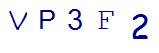# 4 Decimal Math Word Problems Your 5th Grader Should Be Able to SolveLast Updated on May 26, 2022 by Thinkster

Math word problems that include decimals are an important part of 5th-grade math. Students will face more complex math concepts as they transition into middle school, so they must have a strong foundation and be able to solve decimal problems with confidence.

Consider all the ways decimals play an important part in everyday life when creating math word problems, such as budgeting, doing cost comparisons, cooking, and more. Using real-world examples for math word problems is important because kids see and understand the relevance of what they are solving.

The following math word problems are designed to challenge your child to think outside the box with decimals by using simple concepts they can understand.

## 1. Zeroing in on the Zeros

Some decimals include the zero before the point; others don’t. Some might add extra zeros at the end—5.24000 being the same as 5.24.

Fifth graders should be ready for all these instances, as addressed in math word problems like this one:

Michael, Ben, and Kelly are chewing bubble gum. Michael blows a bubble that is 1.340 inches in diameter. Ben’s bubble is .87 inches in diameter. Kelly has a tough time with her bubble, which is only 0.09 inches wide. What is the total diameter of the three bubbles?## 2. Fractions and Decimals

Decimals are simply fractions without obvious numerators or denominators, and fractions are simply decimals that don’t necessarily use base 10.

Here’s a fifth grade math word problem that combines the two:

A barbell that weighs 45.25 pounds is holding 2 plates that weigh 2.2 pounds each and 4 plates that weigh 7½ pounds each. How much weight will Connor be lifting?

To find the answer, students must realize that 7½ is the same as 7.5. Add 45.25, plus (2.2 x 2), plus (7.5 x 4) to get 79.65 pounds.

## 3. Sales Tax

Kids eager to use a \$20 bill to buy something costing \$19.95 often forget about sales tax. Math word problems using sales tax will help them remember:

Allison has \$5 and wants to buy a pack of Pokemon cards that cost \$4.49. The sales tax is 7%. Will she have enough money to buy the cards?

To get the answer, students will need to multiply \$4.49 by 0.07 and then add the result to \$4.49. The rounded answer is \$4.80, so yes, Allison can buy the cards.

## 4. Multiplication, then Subtraction

Here’s another example of multiple operations involving decimals combined into one math word problem.

Assume that your town has no sales tax on food …

Organic cantaloupes cost \$3.99 per melon, and non-organic cantaloupes cost \$2.45 each. If you bought five organic melons, how much more would they cost than five regular ones?To find the answer, the first thing you must do is multiply \$3.99 by 5 to get \$19.95. Then, compute how much five non-organic cantaloupes will cost – \$12.45. From there, simply subtract the lower number from the higher one to get the correct answer of \$7.70.

## Do your kids struggle with decimals or other math word problems?

Word problems can be tricky for students, especially if the math problem has distractors or your child is not comfortable with problem-solving strategies.

Check out this blog for more tips on how to help your child solve either a simple word problem or multi-step word problem?

Consider enrolling them in an online tutoring program, like Thinkster Math.

Our math curriculum includes many critical thinking and logical reasoning problems. We help students build their conceptual understanding skills to help boost their confidence to tackle math word problems.

SummaryArticle Name
4 Decimal Math Word Problems Your 5th Grader Should Be Able to Solve
Description
Is your child able to solve these 4 decimal math word problems? Challenge your child to think outside the box with decimals.
Author
Publisher Name
Thinkster Math
Publisher Logo

## Subscribe to Thinkster Blog## Recommended Articles### Mastering Math: How to Crack Challenging Math Problems in Under a Minute!

Math, often regarded as the king of all sciences, can sometimes pose challenges that leave even the brighte...